Question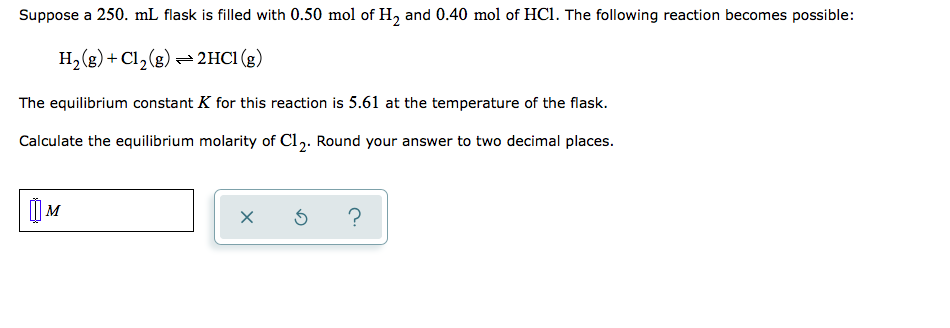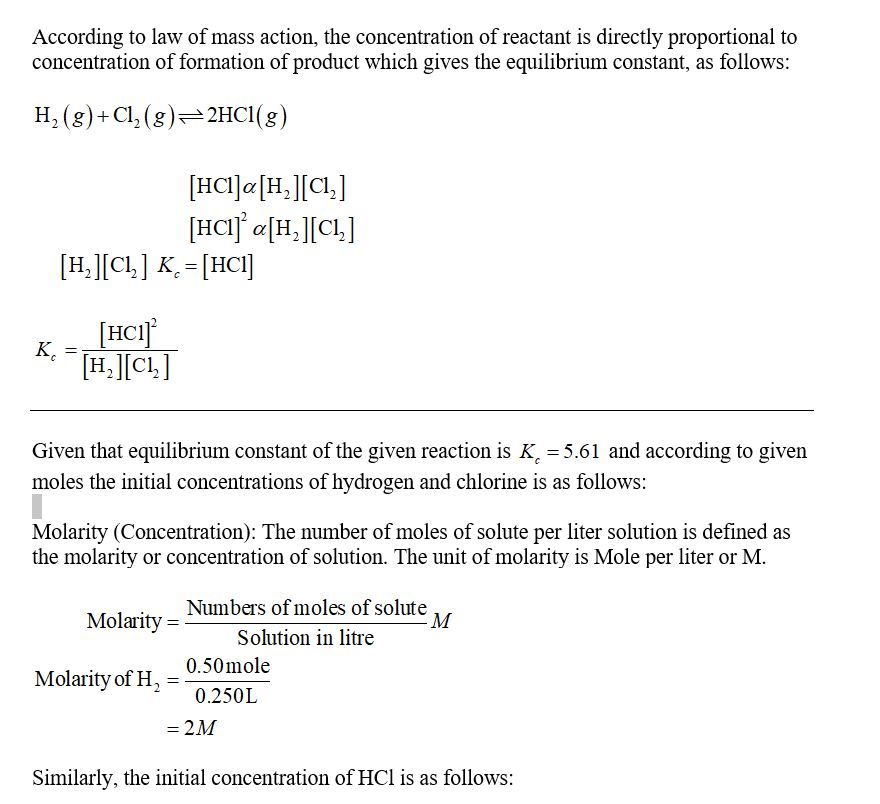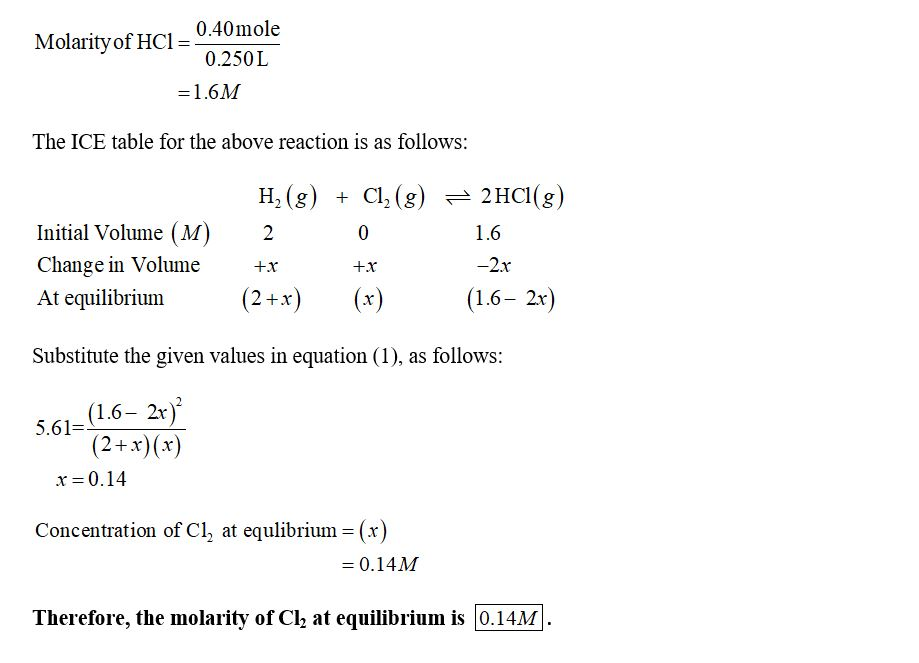#### Earn Coins

Coins can be redeemed for fabulous gifts.

Similar Homework Help Questions
• ### Suppose a 250. ml flask is filled with 1.5 mol of Cl, and 1.3 mol of...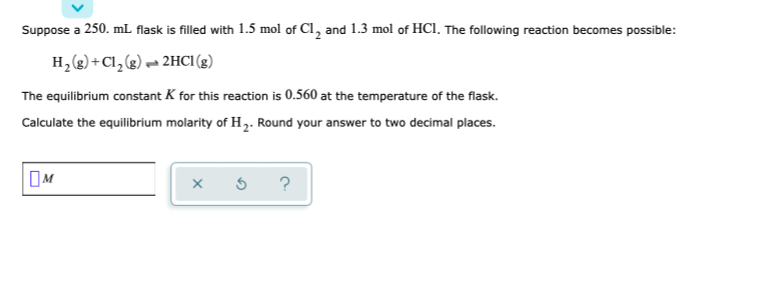Suppose a 250. ml flask is filled with 1.5 mol of Cl, and 1.3 mol of HCl. The following reaction becomes possible: H2(g) + Cl2(g) 2HCl (8) The equilibrium constant K for this reaction is 0.560 at the temperature of the flask. Calculate the equilibrium molarity of H. Round your answer to two decimal places. OM * 5 ?

• ### Suppose a 250. mL flask is filled with 0.10 mol of Cl2 and 1.4 mol of...

Suppose a 250. mL flask is filled with 0.10 mol of Cl2 and 1.4 mol of HCl. The following reaction becomes possible: H2(g)+Cl2(g)=2HCl(g) The equilibrium constant for this reaction is 0.414 at the temperature of the flask. Calculate the equilibrium molarity of . Round your answer to two decimal places.

• ### Suppose a 250. ml flask is filled with 1.7 mol of H, and 0.30 mol of...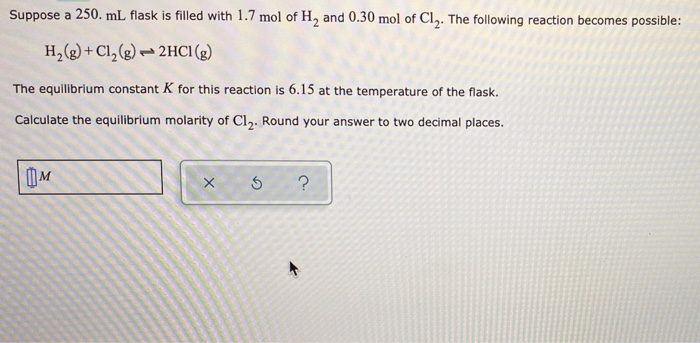Suppose a 250. ml flask is filled with 1.7 mol of H, and 0.30 mol of Cly. The following reaction becomes possible: H2(g) + Cl2(g) - 2HCI(g) The equilibrium constant K for this reaction is 6.15 at the temperature of the flask. Calculate the equilibrium molarity of Cl2. Round your answer to two decimal places. OM xo?

• ### Suppose a 500. ml flask is filled with 1.2 mol of Cl, and 0.80 mol of...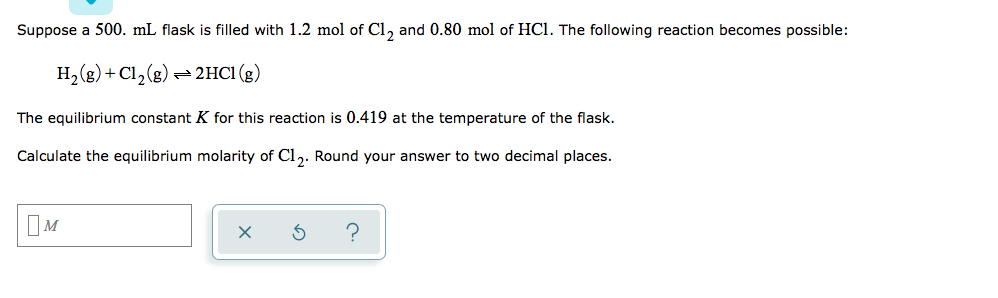Suppose a 500. ml flask is filled with 1.2 mol of Cl, and 0.80 mol of HCl. The following reaction becomes possible: H2(g) + Cl2(g) = 2HCl (g) The equilibrium constant K for this reaction is 0.419 at the temperature of the flask. Calculate the equilibrium molarity of Cl. Round your answer to two decimal places. xs ?

• ### Suppose a 250. ml flask is filled with 0.10 mol of H and 0.30 mol of...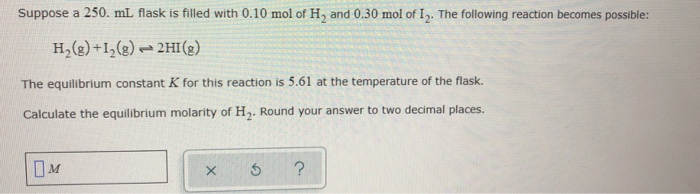Suppose a 250. ml flask is filled with 0.10 mol of H and 0.30 mol of I. The following reaction becomes possible: H2(g) +12(g) - 2HI(g) The equilibrium constant K for this reaction is 5.61 at the temperature of the flask. Calculate the equilibrium molarity of H. Round your answer to two decimal places. OM x ?

• ### Suppose a 250. mL. flask is filled with 1.8 mol of Cl2 and 0.60 mol of...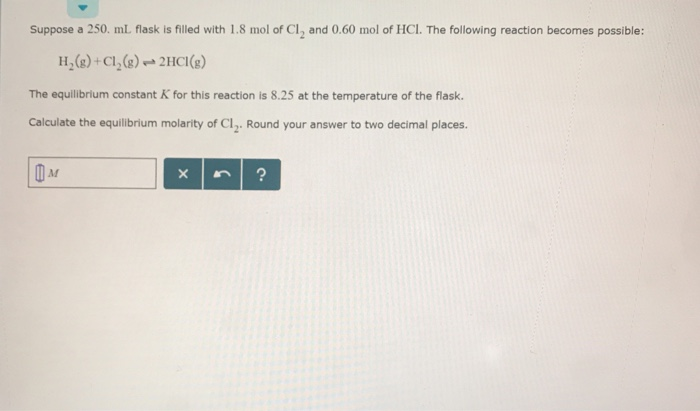Suppose a 250. mL. flask is filled with 1.8 mol of Cl2 and 0.60 mol of HCl. The following reaction becomes possible: H(+Cl()2HCI) The equilibrium constant K for this reaction is 8.25 at the temperature of the flask. Calculate the equilibrium molarity of Cl2. Round your answer to two decimal places.

• ### Suppose a 250. ml flask is filled with 1.6 mol of Cl, and 1.3 mol of...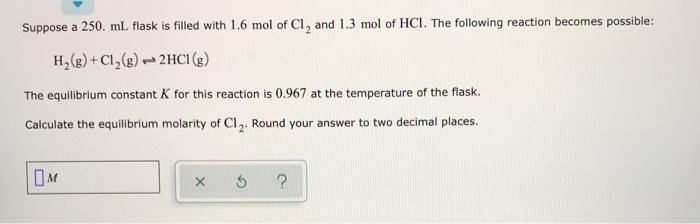Suppose a 250. ml flask is filled with 1.6 mol of Cl, and 1.3 mol of HCI. The following reaction becomes possible: H2(g) + Cl2(g) → 2HCI (g) The equilibrium constant K for this reaction is 0.967 at the temperature of the flask. Calculate the equilibrium molarity of C1. Round your answer to two decimal places. OM I ?

• ### Suppose a 500. ml flask is filled with 1.7 mol of Cl, and 2.0 mol of...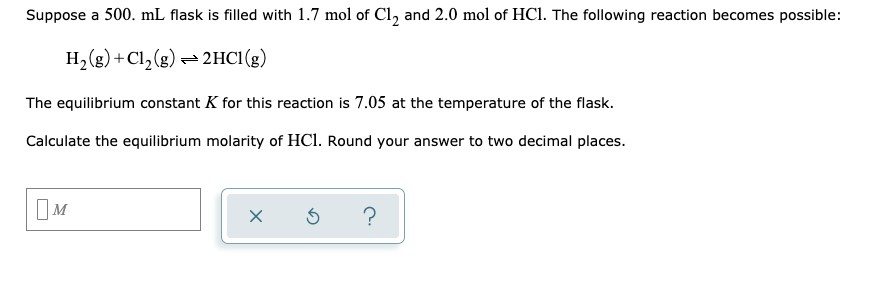Suppose a 500. ml flask is filled with 1.7 mol of Cl, and 2.0 mol of HCl. The following reaction becomes possible: H2(g) +C12(g) + 2HCl (8) The equilibrium constant K for this reaction is 7.05 at the temperature of the flask. Calculate the equilibrium molarity of HCl. Round your answer to two decimal places. x o ?

• ### Suppose a 250. mL flask is filled with 0.50 mol of H20, 1.0 mol of Co2...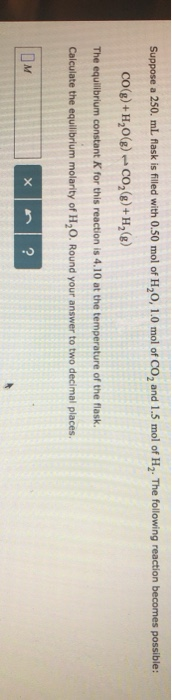Suppose a 250. mL flask is filled with 0.50 mol of H20, 1.0 mol of Co2 and 1.5 mol of H2. The following reaction becomes possible cog)+H20(g)Co()+H28) The equilibrium constant K for this reaction is 4.10 at the temperature of the flask Calculate the equilibrium molarity of H20. Round your answer to two decimal places.

• ### Suppose a 250. mL flask is filled with 1.2 mol of Cl₂

Suppose a 250. mL flask is filled with 1.2 mol of Cl₂, 1.8 mol of CHCl₃ and 0.90 mol of HCl. The following reaction becomes possible:Cl₂(g)+CHCl₃(g) ⇌ HCl(g)+CCl₄(g)The equilibrium constant K for this reaction is 0.855 at the temperature of the flask.Calculate the equilibrium molarity of HCl. Round your answer to two decimal places.